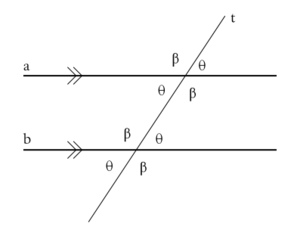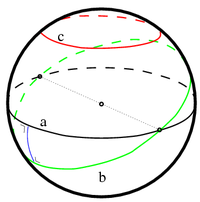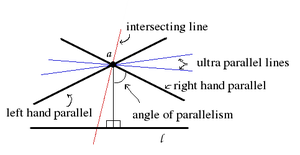# Parallel (geometry)

﻿
Parallel (geometry)

Parallelism is a term in geometry and in everyday life that refers to a property in Euclidean space of two or more lines or planes, or a combination of these. The assumed existence and properties of parallel lines are the basis of Euclid's parallel postulate. Two lines in a plane that do not intersect or touch at a point are called parallel lines. Likewise, a line and a plane, or two planes, in three-dimensional Euclidean space that do not share a point are said to be parallel.

In a non-Euclidean space, parallel lines are those that intersect only in the limit at infinity.

## Symbol

The parallel symbol is$\parallel$ . For example,$AB \parallel CD$ indicates that line AB is parallel to line CD.

In the Unicode character set, the 'parallel' and 'not parallel' signs have codepoints U+2225 (∥) and U+2226 (∦) respectively.

## Euclidean parallelismAs shown by the tick marks, lines a and b are parallel. This can be proved because the transversal t produces congruent angles.

Given straight lines l and m, the following descriptions of line m equivalently define it as parallel to line l in Euclidean space:

1. Every point on line m is located at exactly the same minimum distance from line l (equidistant lines).
2. Line m is on the same plane as line l but does not intersect l (even assuming that lines extend to infinity in either direction).
3. Lines m and l are both intersected by a third straight line (a transversal) in the same plane, and the corresponding angles of intersection with the transversal are equal. (This is equivalent to Euclid's parallel postulate.)

In other words, parallel lines must be located in the same plane, and parallel planes must be located in the same three-dimensional space. A parallel combination of a line and a plane may be located in the same three-dimensional space. Lines parallel to each other have the same gradient. Compare to perpendicular.

### Construction

The three definitions above lead to three different methods of construction of parallel lines.The problem: Draw a line through a parallel to l.

Another definition of parallel line that's often used is that two lines are parallel if they do not intersect, though this definition applies only in the 2-dimensional plane. Another easy way is to remember that a parallel line is a line that has an equal distance with the opposite line.

### Distance between two parallel lines

Because a parallel line is a line that has an equal distance with the opposite line, there is a unique distance between the two parallel lines. Given the equations of two non-vertical parallel lines:$y = mx+b_1\,$$y = mx+b_2\,$

the distance between the two lines can be found by solving the linear system of:$y = mx+b_1\,$$y = -x/m\,$

and the system of:$y = mx+b_2\,$$y = \frac{-x}{m}\,$

to get the coordinates of the points. The solution to the linear systems are:$x_1\ = \frac{-b_1m}{m^2+1}\,$$y_1\ = \frac{b_1}{m^2+1}\,$$x_2\ = \frac{-b_2m}{m^2+1}\,$$y_2\ = \frac{b_2}{m^2+1}\,$

Putting into the distance formula gives us:$d = \sqrt{\left(\frac{b_1m-b_2m}{m^2+1}\right)^2 + \left(\frac{b_2-b_1}{m^2+1}\right)^2}\,$

...$d = \sqrt{\frac{(b_2-b_1)^2}{m^2+1}}\,$$d = \frac{|b_2-b_1|}{\sqrt{m^2+1}}\,$

which of course, is:$d = \frac{|b_2-b_1|}{m^2+1}\sqrt {m^2+1}.$

Also if 2 lines are$ax+by+c_1=0\,$$ax+by+c_2=0\,$

the distance between the two lines can be formulated by the following formula:$d = \frac{|c_2-c_1|}{\sqrt {a^2+b^2}}.$

## Extension to non-Euclidean geometry

In non-Euclidean geometry it is more common to talk about geodesics than (straight) lines. A geodesic is the path that a particle follows if no force is applied to it. In non-Euclidean geometry (spherical or hyperbolic) the three Euclidean definitions are not equivalent: only the second one is useful in other non-Euclidean geometries. In general, equidistant lines are not geodesics so the equidistant definition cannot be used. In the Euclidean plane, when two geodesics (straight lines) are intersected with the same angles by a transversal geodesic (see image), every (non-parallel) geodesic intersects them with the same angles. In both the hyperbolic and spherical plane, this is not the case. For example, geodesics sharing a common perpendicular only do so at one point (hyperbolic space) or at two (antipodal) points (spherical space).

In general geometry it is useful to distinguish the three definitions above as three different types of lines, respectively equidistant lines, parallel geodesics and geodesics sharing a common perpendicular.

While in Euclidean geometry two geodesics can either intersect or be parallel, in general and in hyperbolic space in particular there are three possibilities. Two geodesics can be either:

1. intersecting: they intersect in a common point in the plane
2. parallel: they do not intersect in the plane, but do in the limit to infinity
3. ultra parallel: they do not even intersect in the limit to infinity

In the literature ultra parallel geodesics are often called parallel. Geodesics intersecting at infinity are then called limit geodesics.

### SphericalOn the spherical plane there is no such thing as a parallel line. Line a is a great circle, the equivalent of a straight line in the spherical plane. Line c is equidistant to line a but is not a great circle. It is a parallel of latitude. Line b is another geodesic which intersects a in two antipodal points. They share two common perpendiculars (one shown in blue).

In the spherical plane, all geodesics are great circles. Great circles divide the sphere in two equal hemispheres and all great circles intersect each other. By the above definitions, there are no parallel geodesics to a given geodesic, all geodesics intersect. Equidistant lines on the sphere are called parallels of latitude in analog to latitude lines on a globe. Parallel lines in Euclidean space are straight lines; equidistant lines are not geodesics and therefore are not directly analogous to straight lines in the Euclidean space. An object traveling along such a line has to accelerate away from the geodesic to which it is equidistant to avoid intersecting with it. When embedded in Euclidean space a dimension higher, parallels of latitude can be generated by the intersection of the sphere with a plane parallel to a plane through the center.

### Hyperbolic

In the hyperbolic plane, there are two lines through a given point that intersect a given line in the limit to infinity. While in Euclidean geometry a geodesic intersects its parallels in both directions in the limit to infinity, in hyperbolic geometry both directions have their own line of parallelism. When visualized on a plane a geodesic is said to have a left-handed parallel and a right-handed parallel through a given point. The angle the parallel lines make with the perpendicular from that point to the given line is called the angle of parallelism. The angle of parallelism depends on the distance of the point to the line with respect to the curvature of the space. The angle is also present in the Euclidean case, there it is always 90° so the left and right-handed parallels coincide. The parallel lines divide the set of geodesics through the point in two sets: intersecting geodesics that intersect the given line in the hyperbolic plane, and ultra parallel geodesics that do not intersect even in the limit to infinity (in either direction). In the Euclidean limit the latter set is empty.Intersecting, parallel and ultra parallel lines through a with respect to l in the hyperbolic plane. The parallel lines appear to intersect l just off the image. This is an artifact of the visualisation. It is not possible to isometrically embed the hyperbolic plane in three dimensions. In a real hyperbolic space the lines will get closer to each other and 'touch' in infinity.

Wikimedia Foundation. 2010.

### Look at other dictionaries:

• Parallel — may refer to: Mathematics and science * Parallel (geometry) * Parallel (latitude), an imaginary east west line circling a globe Proper name * Parallel (manga), a shōnen manga by Toshihiko Kobayashi * Parallel (video), a video album by R.E.M. *… …   Wikipedia

• Parallel Lines — For lines that are parallel, see parallel (geometry). Infobox Album Name = Parallel Lines Type = studio Artist = Blondie Released = start date|1978|9 Recorded = June–July 1978 at Record Plant, New York Genre = New Wave Length = 38:11 Label =… …   Wikipedia

• Geometry — (Greek γεωμετρία ; geo = earth, metria = measure) is a part of mathematics concerned with questions of size, shape, and relative position of figures and with properties of space. Geometry is one of the oldest sciences. Initially a body of… …   Wikipedia

• Parallel mesh generation — in numerical analysis is a new research area between the boundaries of two scientific computing disciplines: computational geometry and parallel computingNikos Chrisochoides, Parallel Mesh Generation, Chapter in Numerical Solution of Partial… …   Wikipedia

• Parallel coordinates — is a common way of visualizing high dimensional geometry and analyzing multivariate data. To show a set of points in an n dimensional space, a backdrop is drawn consisting of n parallel lines, typically vertical and equally spaced. A point in n… …   Wikipedia

• geometry — /jee om i tree/, n. 1. the branch of mathematics that deals with the deduction of the properties, measurement, and relationships of points, lines, angles, and figures in space from their defining conditions by means of certain assumed properties… …   Universalium

• Parallel postulate — In geometry, the parallel postulate, also called Euclid s fifth postulate since it is the fifth postulate in Euclid s Elements , is a distinctive axiom in what is now called Euclidean geometry. It states that: If a line segment intersects two… …   Wikipedia

• Parallel transport — In geometry, parallel transport is a way of transporting geometrical data along smooth curves in a manifold. If the manifold is equipped with an affine connection (a covariant derivative or connection on the tangent bundle), then this connection… …   Wikipedia

• Parallel curve — An ellipse (red), its evolute (blue) and some parallel curves (green). Note how the parallel curves have cusps when they touch the evolute …   Wikipedia

• parallel postulate — Geom. the axiom in Euclidean geometry that only one line can be drawn through a given point so that the line is parallel to a given line that does not contain the point. Also called parallel axiom. * * * One of the five postulates, or axioms, of… …   Universalium